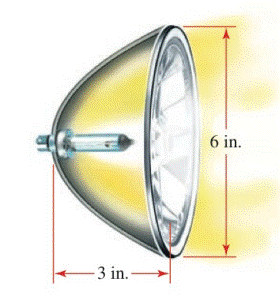Chapter 12.CT, Problem 15CT### Algebra and Trigonometry (MindTap ...

4th Edition
James Stewart + 2 others
ISBN: 9781305071742

#### Solutions

Chapter
Section### Algebra and Trigonometry (MindTap ...

4th Edition
James Stewart + 2 others
ISBN: 9781305071742
Textbook Problem

# A parabolic reflector for a car headlight forms a bowl shape that is 6   in . wide at its opening and 3   in . deep, as shown in the figure at the left. How far from the vertex should the filament of the bulb be placed if it is to be located at the focus?To determine

To find:

The focal point of the parabolic reflector for a car headlight which forms a bowl shape.

Explanation

Given:

The bowl shape parabolic reflector for a car headlight is 6in. wide at its opening and 3in. deep.

Figure 1

Approach:

Introduce a coordinate system and place parabolic reflector in the system such that its vertex is at the origin and 6in. wide side towards the positive x-axis.

Calculation:

Place parabolic reflector in the coordinate system, such that its vertex is at the origin in the positive x-axis, as shown below in the figure. Then the equation of the parabola looks like,

y2=4px

### Still sussing out bartleby?

Check out a sample textbook solution.

See a sample solution

#### The Solution to Your Study Problems

Bartleby provides explanations to thousands of textbook problems written by our experts, many with advanced degrees!

Get Started